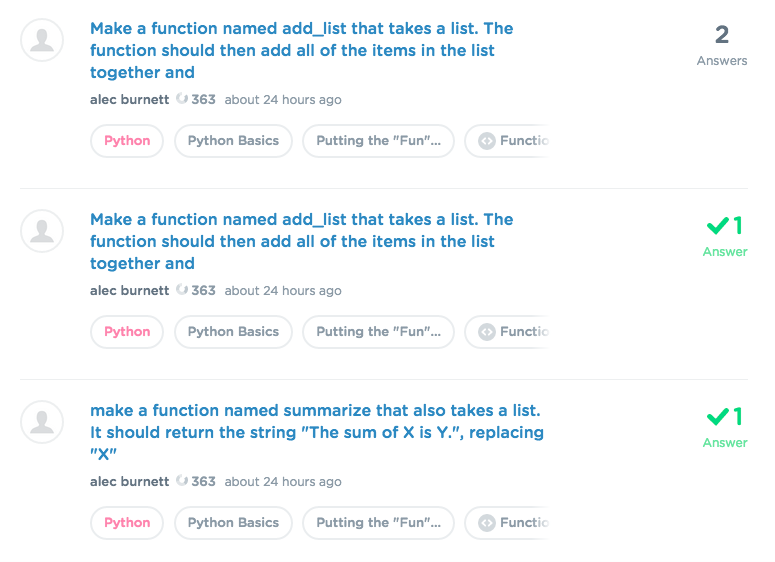## Welcome to the Treehouse Community

Want to collaborate on code errors? Have bugs you need feedback on? Looking for an extra set of eyes on your latest project? Get support with fellow developers, designers, and programmers of all backgrounds and skill levels here with the Treehouse Community!

### Looking to learn something new?

Treehouse offers a seven day free trial for new students. Get access to thousands of hours of content and join thousands of Treehouse students and alumni in the community today.# Make a function named add_list that takes a list. The function should then add all of the items in the list together and

i need help

functions.py
```def add_list(lst):
result = 6
for item in lst:
result += item
return result
```PLUS

Hi alec burnett , I noticed that you've previously posted about this question at least 4 times.And many people have provided answer for in your previous posts, I'd strongly suggest you go check them out instead of continue making new posts. Thanks.

i did non of them are working thats why i keep askingPLUS

OK, let's try to solve this problem once and for all here. :)

So in your codes, there're couple of places need changes.

1. the initial value of result should be 0 instead of 6
2. your indentation of `return result` isn't right, it should be indented outside of the for loop, that way you can return result 's value after the for loop is finished running.
```def add_list(lst):
result = 0
for item in lst:
result += item
return result
```

i just tried that and it didn't work

ok thank you William lee that worked now my friend told me the second part but it didn't work can you help me there as well

how do i show the code sorry im new to this treehouse thing lol

```def add_list(lst) :
result = 0
for item in lst:
result += item
return result
def summarize (another_list) :
return "The sum of {} is.".format(' '.join(another_list), sum(another_list))
```

here you go william leePLUS

Hello, alec burnett

You got alot of it correct in your code :P. There're some places need changes. First, take another look of what the challenge is asking you to do

It should return the string "The sum of X is Y.", replacing "X" with the string version of the list and "Y" with the sum total of the list.

##### summarize([1, 2, 3]) should return "The sum of [1, 2, 3] is 6."

So in the return String, there're two places you need to insert values into, therefore, you need 2 {} inside your return String, not just 1.

• the first {}, should be just the argument another_list.
• the second {}, should be the sum of the list, which you got it correct, sum(another_list) is the way to go.

And the final code should be.

```def add_list(lst):
result = 0
for item in lst:
result += item
return result

def summarize (another_list) :
return "The sum of {} is {}.".format(another_list, sum(another_list))
```

Hope you find it useful, if you have more question, feel free to post it here.I haven't check what is asked but I can tell that your `return` is not placed at the right spot. Currently this `return` will end your function after one iteration of the loop. That's not what you want here.

```def add_list(lst):
result = 6
for item in lst:
result += item

return result
```